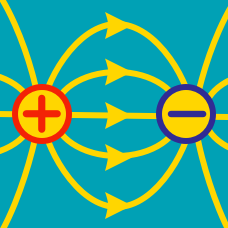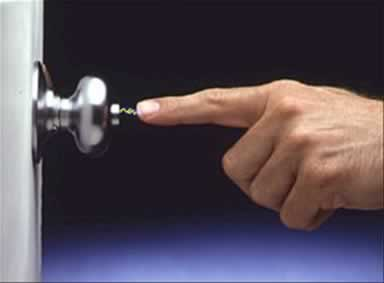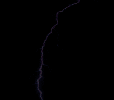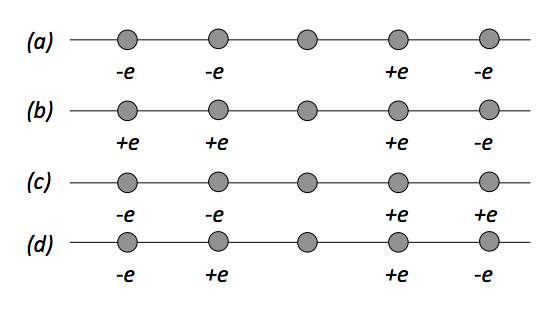Electricity and Magnetism

# Electric Fields: Level 2-3 ChallengesAfter scraping your feet against a carpet or rug, you often get a shock when touching a metal object. This shock is due to which of the following?Lightning occurs when there is a breakdown in the electrical insulating properties of air. That is, the potential difference between the ground and a cloud is so great that it overcomes the fact that air usually a pretty good insulator. Air begins to conduct at a potential difference of approximately $3 \times 10^6\text{ V/m}$.

A typical height for a cloud to ground lightning strike is 1 km.

What is the total voltage difference between the cloud and the ground right before the lightning strike in Volts?

###### Image credit: WikipediaThree identical conducting spheres are located at the vertices of an equilateral triangle $ABC.$ Initially the charge of the sphere at point $A$ is $q_{A}=0$ and the spheres at $B$ and $C$ carry the same charge $q_{B}=q_{C}=q.$ It is known that the sphere $B$ exerts an electrostatic force on $C$ which has a magnitude $F=4~\mbox{N}.$ Suppose an engineer connects a very thin conducting wire between spheres $A$ and $B.$ Then she removes the wire and connects it between spheres $A$ and $C.$ After these operations, what is the magnitude of the force of interaction in Newtons between $B$ and $C?$

A thin disc of radius $\displaystyle R = 10 \text{ cm }$made of non-conducting material is charged uniformly so as to acquire a uniform surface charge density $\displaystyle \sigma = 5 \text{ nC}/\text{m}^2$.

Find the potential at the edge of the disc in Volts.

Details and Assumptions

• $\displaystyle \frac{1}{4 \pi \epsilon _{0} } = 9 \times 10^{9} \text{ N}\cdot \text{m}^2 / \text{C}^2$The figures above show four situations in which five charged particles are evenly placed along an axis. The charge values are known except for the particle in the center, which has the same charge across all four situations. Which situation gives the greatest magnitude of net electrostatic force on the particle in the center?

×